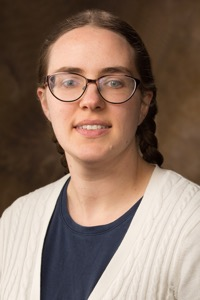## Ariel Barton’s Homepage

### Contact Information

Office: SCEN 222
Email: aeb019@uark.edu

### Office Hours

Wednesdays 2:00, Thursdays 1:00, Fridays 3:00, or by appointment, SCEN 222

### Research Interests

Partial Differential Equations
Harmonic Analysis### Courses

 (Fall 2021) Math 4513/5213; Math 5503 (Spring 2021) Math 2584C; Math 4523/5223 (Fall 2020) Math 4513/5213 (Spring 2020) Math 2584C; Math 4513/5213 (Fall 2019) Math 2584C (Spring 2019) Math 3083 (Fall 2018) Math 2554H; Math 5313 (Spring 2018) Math 2584C (Fall 2017) Math 2584C; Math 2574H (Fall 2016) Math 2584C; Math 5533 (Spring 2016) Math 2584C; Math 5523

### Papers and preprints

“The Lp Neumann problem for higher order elliptic equations” (PDF file), submitted. 55 pages.

“The W −1, p Neumann problem for higher order elliptic equations” (PDF file), submitted. 47 pages.

(with Steve Hofmann and Svitlana Mayboroda) “Nontangential estimates on layer potentials and the Neumann problem for higher order elliptic equations” (PDF file). Int. Math. Res. Not. IMRN (2020), https://doi.org/10.1093/imrn/rnaa051. 49 pages.

(with Steve Hofmann and Svitlana Mayboroda) “The Neumann problem for higher order elliptic equations with symmetric coefficients” (PDF file), Math. Ann. 371 (2018), no. 1–2. 34 pages.

(with Steve Hofmann and Svitlana Mayboroda) “Dirichlet and Neumann boundary values of solutions to higher order elliptic equations” (PDF file), Ann. Inst. Fourier (Grenoble) 69 (2019), no. 4. 42 pages.

(with Steve Hofmann and Svitlana Mayboroda) “Bounds on layer potentials with rough inputs for higher order elliptic equations” (PDF file), Proc. Lond. Math. Soc. (3) 119 (2019), no. 3. 44 pages.

“Trace and extension theorems relating Besov spaces to weighted averaged Sobolev spaces” (PDF file), Math. Inequal. Appl. 21 (2018), no. 3. 48 pages.

(with Steve Hofmann and Svitlana Mayboroda) “Square function estimates on layer potentials for higher-order elliptic equations” (PDF file), Math. Nachr. 290 (2017), no. 16. 62 pages.

“Layer potentials for general linear elliptic systems” (PDF file), Electron. J. Differential Equations 2017 (2017), no. 309. 23 pages.

“Gradient estimates and the fundamental solution for higher-order elliptic systems with rough coefficients” (PDF file), Manuscripta Math. 151 (2016), no. 3–4. 41 pages.

(with Svitlana Mayboroda) “Layer potentials and boundary-value problems for second order elliptic operators with data in Besov spaces” (PDF file), Mem. Amer. Math. Soc. 243 (2016), no. 1149. 109 pages, 17 figures. There is also a version with colored figures.

(with Svitlana Mayboroda) “Higher-order elliptic equations in non-smooth domains: a partial survey” (PDF file), Harmonic analysis, partial differential equations, complex analysis, Banach spaces, and operator theory. Vol. 1, 55–121, Assoc. Women Math. Ser., 4, Springer, [Cham], 2016. 56 pages. An earlier version appeared in: Concrete operators, spectral theory, operators in harmonic analysis and approximation, Oper. Theory Adv. Appl., vol. 236, Birkhäuser/Springer, Basel, 2014. 41 pages.

“The Dirichlet problem with BMO boundary data and almost-real coefficients” (PDF file), Rev. Mat. Iberoam. 31 (2015), no. 2. 29 pages.

(with Lesley Ward) “A new class of harmonic measure distribution functions” (PDF file), J. Geom. Anal. 24 (2014), no. 4. 34 pages, 7 figures.

(with Svitlana Mayboroda) “The Dirichlet problem for higher order equations in composition form” (PDF file). J. Funct. Anal. 265 (2013), no. 1. 51 pages.

“Elliptic partial differential equations with almost-real coefficients” (PDF file). Mem. Amer. Math. Soc. 223 (2013), no. 1051. 108 pages, 5 figures.

### Documents

My curriculum vita (PDF file)
Exam timer
Direction field plotter
Phase plane plotter